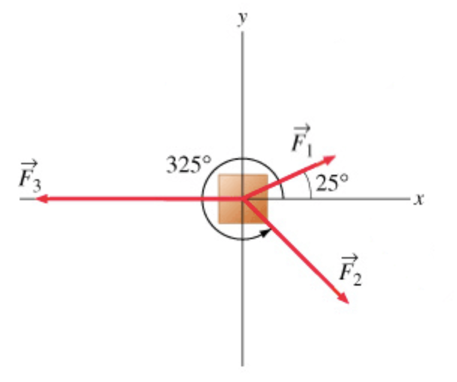# Problem: The diagram below shows a block of mass m = 2.00 kg on a frictionless horizontal surface, as seen from above. Three forces of magnitudes F4.00 N, F2 6.00 N, and F3 8.00 N are applied to the block, initially at rest on the surface, at angles shown on the diagram. (Figure 1) In this problem, you will determine the resultant (total) force vector from the combination of the three individual force vectors. All angles should be measured counterclockwise from the positive x axis (i.e., all angles are positive).

###### FREE Expert Solution

A force can be expressed as:

$\overline{)\stackrel{\mathbf{⇀}}{\mathbf{F}}{\mathbf{=}}{\mathbf{|}}{\mathbf{F}}{\mathbf{|}}{\mathbf{c}}{\mathbf{o}}{\mathbf{s}}{\mathbf{\theta }}\stackrel{\mathbf{^}}{\mathbf{i}}{\mathbf{+}}{\mathbf{|}}{\mathbf{F}}{\mathbf{|}}{\mathbf{s}}{\mathbf{i}}{\mathbf{n}}{\mathbf{\theta }}\stackrel{\mathbf{^}}{\mathbf{j}}}$

Magnitude:

$\overline{){\mathbf{|}}{\mathbf{F}}{\mathbf{|}}{\mathbf{=}}\sqrt{{{\mathbf{F}}_{\mathbf{x}}}^{\mathbf{2}}\mathbf{+}{{\mathbf{F}}_{\mathbf{y}}}^{\mathbf{2}}}}$

For the three forces, we have:

96% (253 ratings)###### Problem Details

The diagram below shows a block of mass m = 2.00 kg on a frictionless horizontal surface, as seen from above. Three forces of magnitudes F4.00 N, F2 6.00 N, and F3 8.00 N are applied to the block, initially at rest on the surface, at angles shown on the diagram. (Figure 1) In this problem, you will determine the resultant (total) force vector from the combination of the three individual force vectors. All angles should be measured counterclockwise from the positive x axis (i.e., all angles are positive).